MATLAB Function Referenceerf, erfc, erfcx, erfinv, erfcinv

Error functions

Syntax

• ```Y = erf(X)             Error function
Y = erfc(X)            Complementary error function
Y = erfcx(X)           Scaled complementary error function
X = erfinv(Y)          Inverse error function
X = erfcinv(Y)         Inverse complementary error function
```

Definition

The error function `erf(X)` is twice the integral of the Gaussian distribution with 0 mean and variance of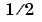.

•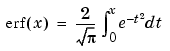The complementary error function `erfc(X)` is defined as

•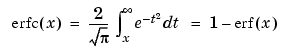The scaled complementary error function `erfcx(X)` is defined as

•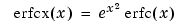For large `X`, `erfcx(X)` is approximately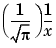Description

```Y = erf(X) ``` returns the value of the error function for each element of real array `X`.

```Y = erfc(X) ``` computes the value of the complementary error function.

```Y = erfcx(X) ``` computes the value of the scaled complementary error function.

```X = erfinv(Y) ``` returns the value of the inverse error function for each element of `Y`. Elements of `Y` must be in the interval `[-1 1]`. The function `erfinv` satisfies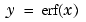forand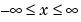.

```X = erfcinv(Y) ``` returns the value of the inverse of the complementary error function for each element of `Y`. Elements of `Y` must be in the interval `[0 2]`. The function `erfcinv` satisfies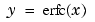for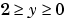and.

Remarks

The relationship between the complementary error function `erfc` and the standard normal probability distribution returned by the Statistics Toolbox function `normcdf` is

•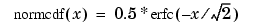The relationship between the inverse complementary error function `erfcinv` and the inverse standard normal probability distribution returned by the Statistics Toolbox function `norminv` is

•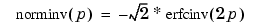Examples

`erfinv(1)` is `Inf`

`erfinv(-1)` is `-Inf`.

For `abs(Y)` `>` `1`, `erfinv(Y)` is `NaN`.

Algorithms

For the error functions, the MATLAB code is a translation of a Fortran program by W. J. Cody, Argonne National Laboratory, NETLIB/SPECFUN, March 19, 1990. The main computation evaluates near-minimax rational approximations from .

For the inverse of the error function, rational approximations accurate to approximately six significant digits are used to generate an initial approximation, which is then improved to full accuracy by one step of Halley's method.

References

  Cody, W. J., "Rational Chebyshev Approximations for the Error Function," Math. Comp., pgs. 631-638, 1969

© 1994-2005 The MathWorks, Inc.